Denjoy integral

(diff) ← Older revision | Latest revision (diff) | Newer revision → (diff)

The narrow (special) Denjoy integral is a generalization of the Lebesgue integral. A functionis said to be integrable in the sense of the narrow (special,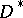) Denjoy integral onif there exists a continuous functiononsuch that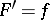almost everywhere, and if for any perfect setthere exists a portion ofon whichis absolutely continuous and where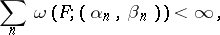where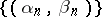is the totality of intervals contiguous to that portion ofand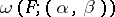is the oscillation ofon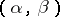;This generalization of the Lebesgue integral was introduced by A. Denjoy

who showed that his integral reproduces the function with respect to its pointwise finite derivative. Theintegral is equivalent to the Perron integral.

The wide (general) Denjoy integral is a generalization of the narrow Denjoy integral. A functionis said to be integrable in the sense of the wide (general,) Denjoy integral onif there exists a continuous functiononsuch that its approximate derivative is almost everywhere equal toand if, for any perfect set, there exists a portion ofon whichis absolutely continuous; hereIntroduced independently, and almost at the same time, by Denjoy

and A.Ya. Khinchin , . Theintegral reproduces a continuous function with respect to its pointwise finite approximate derivative.

A totalizationis a constructively defined integral for solving the problem of constructing a generalized Lebesgue integral which would permit one to treat any convergent trigonometric series as a Fourier series (with respect to this integral). Introduced by Denjoy .

A totalization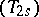differs from a totalizationby the fact that the definition of the latter totalization involves an approximate rather than an ordinary limit. Denjoy  also gave a descriptive definition of a totalization. For relations between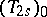andand other integrals, see .

How to Cite This Entry:
Denjoy integral. Encyclopedia of Mathematics. URL: http://encyclopediaofmath.org/index.php?title=Denjoy_integral&oldid=12910
This article was adapted from an original article by T.P. Lukashenko (originator), which appeared in Encyclopedia of Mathematics - ISBN 1402006098. See original article